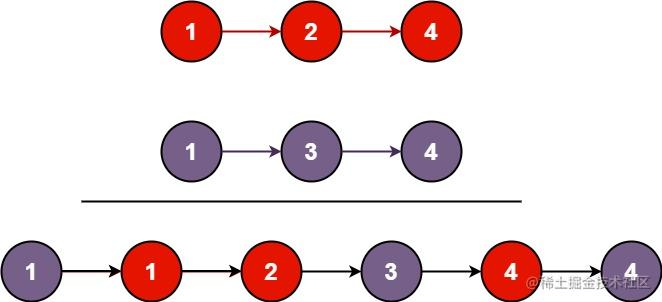# 前端刷题路-Day56：合并两个有序链表（题号21）

### 合并两个有序链表（题号21）

#### 题目``````输入：l1 = [1,2,4], l2 = [1,3,4]

``````输入：l1 = [], l2 = []

``````输入：l1 = [], l2 = 

• 两个链表的节点数目范围是 `[0, 50]`
• `-100 <= Node.val <= 100`
• `l1``l2` 均按 非递减顺序 排列

#### 链接

leetcode-cn.com/problems/me…

#### 解释

1. 有一条链表没了怎么办
2. 两条链表的节点插入顺序是什么

#### 自己的答案（迭代）

``````var mergeTwoLists = function(l1, l2) {
while (l1 || l2) {
if ((!l1 && l2) || l2 && l1.val >= l2.val) {
node.next = l2
node = node.next
l2 = l2.next
} else {
node.next = l1
node = node.next
l1 = l1.next
}
}
};

#### 更好的方法（迭代）

``````var mergeTwoLists = function(l1, l2) {
while (l1 && l2) {
if (l1.val < l2.val) {
node.next = l1
l1 = l1.next
} else {
node.next = l2
l2 = l2.next
}
node = node.next
}
node.next = l1 ? l1 : l2
};

#### 更好的方法（递归）

``````var mergeTwoLists = function(l1, l2) {
if (!l1 && !l2) return null
if (!l1) {
return l2
} else if (!l2) {
return l1
} else if (l1.val >= l2.val) {
l2.next = mergeTwoLists(l1, l2.next)
return l2
} else {
l1.next = mergeTwoLists(l2, l1.next)
return l1
}
};PS：想查看往期文章和题目可以点击下面的链接：

Here is RZ